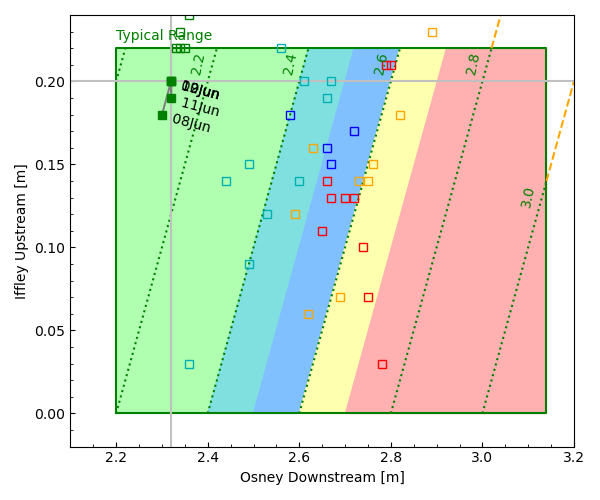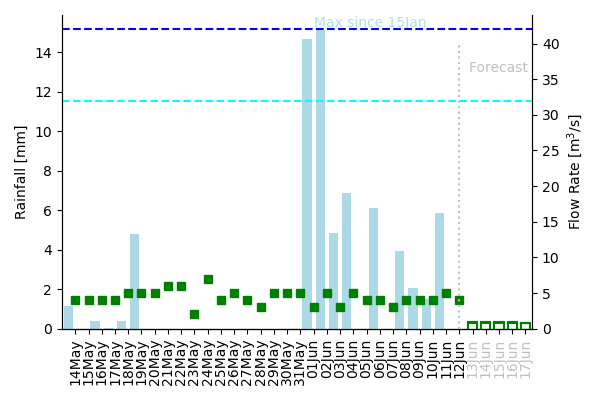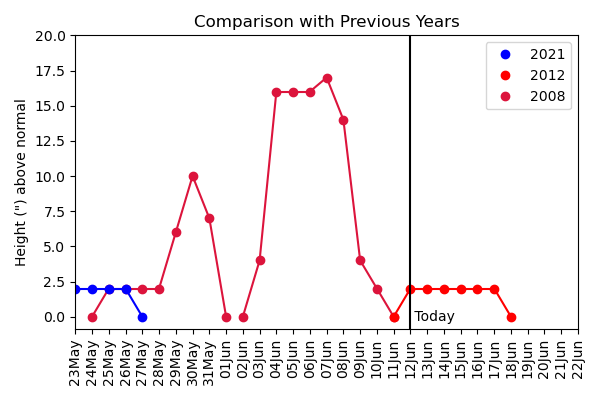# Isis Level

• 27 June: (14:16) Grey flag - no more official flag updates over the summer
• 13 May: (15:00) Green Flag
• 12 May: (12:24) Light Blue Flag
• 09 May: (13:01) Dark Blue Flag
• 28 Apr: (14:51) Green Flag
• 26 Apr: (15:47) Light Blue Flag
• 21 Apr: (17:29) Green Flag

 Isis Flag: [Status] The river level and towpath flooding plots continue to be updated. EA Boards: Osney Lock No lock transit on Red or Yellow boards (OURCs Rule Rule 2.6.n) [Latest] Iffley Lock (Status at 06:30 1 June)

### EA Gauges

The plot below shows recent levels recorded by the EA gauges at the upstream end of Iffley lock (IU) and the downstream end of Osney Lock (OD), which mark the lower and upper ends of the main part of the Isis within Oxford.The green-bordered rectangle shows region within the EA definition of the 'Typical Range' for both gauges, with coloured bands indicating approximate ranges of different flag conditions (assuming that the flow speed is related to the OD-IU difference). Dotted lines are lines of constant (OD-IU) difference, i.e. approximately constant flow speed.

Solid squares, with dates, show the last 5 days, open squares are the most recent data for each flag type.

### Flow Rate & Rainfall

The Osney-Iffley river level difference, which controls the flow speed (distance/time) can vary rapidly from day-to-day as the weirs are opened or closed, but a more smoothly varying quantity is the flow rate (volume/time), which is the product of flow speed x cross-section area. This is expected to increase rapidly following heavy rainfall (after a delay of about a day or two), and then smoothly decay back to a 'normal' level.The plot above shows the past 30 days of estimated flow rate (m3/s, left-axis, squares), derived as below, and daily rainfall (mm, right-axis, bars), obtained from the AOPP Weather Station in central Oxford.

Flow rate F [m3/s] is estimated from

F = 100 ( OD - IU - 2.23 )
where OD and IU are the Osney Downstream and Iffley Upstream measurements (in metres).

The number 2.23 is chosen to give approx zero flow for the OD-IU measurements during dry summer conditions (this ought to match the nominal altitude difference 2.15m between the two locks, but this give an unrealistic flow). The factor 100 is chosen to convert to a flow rate which approximately matches the geometric average of the nearest Thames flow meters which are about 10 miles upstream (Farmoor) and, while it was working, 10 miles dowstream (Sutton Courtenay).

The Forecast assumes no further rain, and that the flow F(i) [m3/s] for day i can be modelled as

F(i) = B + A * R(i-2) + F(i-1) * exp(-1/T)
where F(i-1) is the previous day's flow, R(i-2) is the rainfall [mm] from two days previously. A (response), B (background flow), T (time constant) are parameters obtained from the best fit to the previous month's data (current values shown on the graph) and the error bars represent the RMS error with which this function fits the previous month's data.